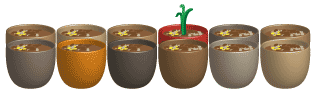## Content description

List outcomes of chance experiments involving equally likely outcomes and represent probabilities of those outcomes using fractions (ACMSP116)
Recognise that probabilities range from 0 to 1 (ACMSP117)

Source: Australian Curriculum, Assessment and Reporting Authority (ACARA)

### Assigning probabilities to outcomes

Many experiments we do in probability involve the use of dice. The singular of dice is die, so we can roll one die or many dice. We can list the outcomes when we roll a single die just once:

1, 2, 3, 4, 5 or 6.

The chance of rolling any number between one and six is 'equally likely'. That means that there is as much chance of rolling a 2 as there is of rolling a 5 on one roll of a single die.

The probability that a 5 will be rolled is just one outcome out of six possible outcomes. We write 'one out of six' as the fraction $$\dfrac{1}{6}$$ and we say that the probability of rolling a 5 on a normal six-sided die is 'one sixth'.Detailed description

Outcomes have a probability between 0 and 1, and are often written as fractions.

If we spin this spinner once and observe the colour, there are four equally likely outcomes. They are orange, red, green or blue. The probability of each outcome is $$\dfrac{1}{4}$$.

For a chance experiment with five equally likely outcomes, the probability of each of these outcomes is $$\dfrac{1}{5}$$.

The probability that the seedling pictured would be the first to sprout is $$\dfrac{1}{12}$$ because there are exactly 12 seeds, one in each little pot, so there are 12 outcomes.